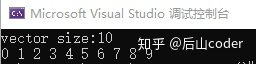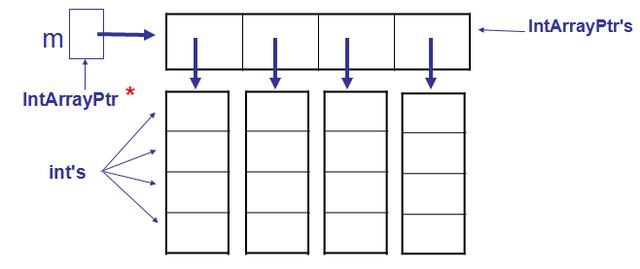• ## c动态数组

千次阅读 2013-11-24 00:20:58
在创建动态数组的过程中我们要遵循一个原则，那就是在创建的时候从外层往里层，逐层创建；而释放的时候从里层往外层，逐层释放。这个话你读了可能理解并不深刻，不过不要急，接下来我们看看两段代码。 一维动态数组...
在创建动态数组的过程中我们要遵循一个原则，那就是在创建的时候从外层往里层，逐层创建；而释放的时候从里层往外层，逐层释放。这个话你读了可能理解并不深刻，不过不要急，接下来我们看看两段代码。
一维动态数组的创建：
#include <stdio.h>
#include <stdlib.h>
int main()
{
int n1,i;
int *array;
printf("请输入所要创建的一维动态数组的长度：");
scanf("%d",&n1);
array=(int*)calloc(n1,sizeof(int));
for(i=0;i<n1;i++)
{
printf("%d\t",array[i]);
}
printf("\n");
for(i=0;i<n1;i++)
{
array[i]=i+1;
printf("%d\t",array[i]);
}
free(array);//释放第一维指针
return 0;
}
运行结果为：特此说明：在以后的运行结果部分，我均会附上文字结果，以防图片打开失败。
请输入所要创建的一维动态数组的长度：4
0       0       0       0
1       2       3       4       Press any key to continue
在此我使用的是calloc()函数来分配的，同时也使用两个for语句来打印数组元素，我们发现第一个打印输出的数组元素值均为0，在此也是为了加深读者对于calloc()函数的印象我特地使用了它来分配，如果对于calloc()、malloc()、realloc()函数的区别还是很清楚的读者可以去看看我的另外一篇博客------C语言的那些小秘密之内存分配。
二维数组的创建：
#include <stdio.h>
#include <stdlib.h>
int main()
{
int n1,n2;
int **array,i,j;
printf("请输入所要创建的动态数组的第一维长度：");
scanf("%d",&n1);
printf("请输入所要创建的动态数组的第二维长度：");
scanf("%d",&n2);
array=(int**)malloc(n1*sizeof(int*)); //第一维
for(i=0;i<n1; i++)
{
array[i]=(int*)malloc(n2* sizeof(int));//第二维
}
for(i=0;i<n1;i++)
{
for(j=0;j<n2;j++)
{
array[i][j]=i*n2+j+1;
printf("%d\t",array[i][j]);
}
printf("\n");
}
for(i=0;i<n1;i++)
{
free(array[i]);//释放第二维指针
}
free(array);//释放第一维指针
return 0;
}
运行结果为：请输入所要创建的动态数组的第一维长度：3
请输入所要创建的动态数组的第二维长度：3
1       2       3
4       5       6
7       8       9
Press any key to continue
有了上面的代码我们再来说动态数组的建立就简单了，以二维为例，先说创建，还记得我们上面说的创建的原则嘛：从外层往里层，逐层创建。
array=(int**)malloc(n1*sizeof(int*)); //第一维
以上是我们创建二维动态数组的最外层，创建好了最外层那么我们接下来就是要创建次外层了。
array[i]=(int*)malloc(n2* sizeof(int));//第二维
在创建次外层的过程中我们使用了一个for喜欢语句，千万别忘了使用for循环语句，这是绝大多数人的一个易错点。
创建好了接下来我们该讲到释放了，而释放的时候从里层往外层，逐层释放。刚刚与我们上面的创建相反，在以上代码中我们首先使用了下面一个for循环来释放里层。
for(i=0;i<n1;i++)
{
free(array[i]);//释放第二维指针
}
在通过以下语句来释放外层。
free(array);//释放第一维指针
如果出现多维的情况怎么做呢，我们接下来再来看看一个三维动态数组的创建和释放，以加深下读者的印象。代码如下：
#include <stdlib.h>
#include <stdio.h>
int main()
{
int n1,n2,n3;
int ***array;
int i,j,k;
printf("请输入所要创建的动态数组的第一维长度：");
scanf("%d",&n1);
printf("请输入所要创建的动态数组的第二维长度：");
scanf("%d",&n2);
printf("请输入所要创建的动态数组的第三维长度：");
scanf("%d",&n3);
array=(int***)malloc(n1*sizeof(int**));//第一维
for(i=0; i<n1; i++)
{
array[i]=(int**)malloc(n2*sizeof(int*)); //第二维
for(j=0;j<n2;j++)
{
array[i][j]=(int*)malloc(n3*sizeof(int)); //第三维
}
}
for(i=0;i<n1;i++)
{
for(j=0;j<n2;j++)
{
for(k=0;k<n3;k++)
{
array[i][j][k]=i+j+k+1;
printf("%d\t",array[i][j][k]);
}
printf("\n");
}
printf("\n");
}
for(i=0;i<n1;i++)
{
for(j=0;j<n2;j++)
{
free(array[i][j]);//释放第三维指针
}
}
for(i=0;i<n1;i++)
{
free(array[i]);//释放第二维指针
}
free(array);//释放第一维指针
return 0;
}
运行结果为：请输入所要创建的动态数组的第一维长度：3
请输入所要创建的动态数组的第二维长度：3
请输入所要创建的动态数组的第三维长度：3
1       2       3
2       3       4
3       4       5
2       3       4
3       4       5
4       5       6
3       4       5
4       5       6
5       6       7
Press any key to continue
看了以上三维动态数组的创建和释放代码以后，我想读者这个时候已经可以自己编写任意维的动态数组了。但是细心的读者可能发现了一个问题，那就是我们所讲的动态数组都是一次性创建好的，如果接下来在使用的过程中我们使用的数组需要扩展或者删减一些不再使用元素该怎么办呢？！接下来我们先看一段关于动态数组扩展的代码，在此以一维动态数组的扩展为例，其它的以此类推。
#include <stdio.h>
#include <stdlib.h>
int main()
{
int*n,*p;
int i,n1,n2;
printf("请输入所要创建的动态数组的长度：");
scanf("%d",&n1);
n=(int*)calloc(n1,sizeof(int));
printf("请输入所要扩展的动态数组的长度：");
scanf("%d",&n2);
p=(int*)realloc(n,(n2)*sizeof(int));//动态扩充数组
for(i=0;i<n2;i++)
{
p[i]=i+1;
if(i%5==0)
printf("\n");
printf("%d\t",p[i]);
}
free(p);
return 0;
}
运行结果如下：请输入所要创建的动态数组的长度：6
请输入所要扩展的动态数组的长度：25
1       2       3       4       5
6       7       8       9       10
11      12      13      14      15
16      17      18      19      20
21      22      23      24      25      Press any key to continue
看了上面的代码读者应该知道如何来扩展动态数组了，可能有的读者对于realloc()函数的使用有些陌生，如果有什么疑惑的话可以参考我之前写的一篇博文------C语言的那些小秘密之内存分配，在此我就不再做过多的讲解了。
接下来如何缩小动态数组。
#include <stdio.h>
#include <stdlib.h>
int main()
{
int*n,*p;
int i,n1,n2;
printf("请输入所要创建的动态数组的长度：");
scanf("%d",&n1);
n=(int*)calloc(n1,sizeof(int));
for(i=0;i<n1;i++)
{
n[i]=i+1;
if(i%5==0)
printf("\n");
printf("%d\t",n[i]);
}
printf("\n请输入所要缩小的动态数组的长度：");
scanf("%d",&n2);
p=(int*)realloc(n,(n2)*sizeof(int));
for(i=0;i<n2;i++)
{
if(i%5==0)
printf("\n");
printf("%d\t",p[i]);
}
printf("\n");
free(p);
return 0;
}
运行结果为：请输入所要创建的动态数组的长度：25
1       2       3       4       5
6       7       8       9       10
11      12      13      14      15
16      17      18      19      20
21      22      23      24      25
请输入所要缩小的动态数组的长度：15
1       2       3       4       5
6       7       8       9       10
11      12      13      14      15
Press any key to continue
在这里值得注意的一点就是在缩减动态数组的时候，它是删除了后面的元素，而前面的元素保持不变。在使用realloc()函数的时候要由其注意它的使用规则。

展开全文• liunx c动态数组 功能 1.对数组初始化 2. 检测数组是否为空 3. 遍历数组 4. 检测数组存储满了 5. 追加数据 6. 指定位置插入数据 7. 删除指定位置的数据并返回该数字 8. 倒置 9. 数组排序 代码 #include <stdio.h&...
liunx c动态数组
功能
1.对数组初始化
2. 检测数组是否为空
3. 遍历数组
4. 检测数组存储满了
5. 追加数据
6. 指定位置插入数据
7. 删除指定位置的数据并返回该数字
8. 倒置
9. 数组排序
代码
#include <stdio.h>
#include <string.h>
#include <malloc.h>
#include <stdlib.h>
typedef struct Array
{
int * data;  //用于存储内容
int len;      //存放的个数
int count;    //当前数据的个数
} Arr;
void init_arr(struct Array * p,const int len);  //对data初始化
bool is_empty(struct Array * p);                //检测count是否为0
void show_arr(struct Array * p);                //输出data
bool full_arr(struct Array * p);                //检测len是否等于count
bool add_arr(struct Array * p,const int val);   //追加内容
bool insert_arr(struct Array * p,const int pos,const int val); //指定位置插入数字
bool del_arr(struct Array * p,const int pos,int * re);         //删除指定位置的数字,然后然后返所删除的数字
bool inversion_arr(struct Array * p);  //倒置
bool sort_arr(struct Array * p); //排序
int main()
{
Arr arr;
init_arr(&arr,10);
sort_arr(&arr);
show_arr(&arr);
return 0;
}
void init_arr(struct Array * p,const int len)
{
p->data = (int *)malloc(sizeof(int) * len);
if(p->data == NULL)
{
exit(-1);
}
else
{
p->len = len;
p->count = 0;
}
return;
}
bool is_empty(struct Array * p)
{
if(p->count == 0)
return true;
else
return false;
}
void show_arr(struct Array * p)
{
if(is_empty§ == true) printf(“数组为空\n”);
else
{
for(int i = 0; i < p->count; i++)
{
printf("%d\n",p->data[i]);
}
}
}
bool add_arr(struct Array * p,const int val)
{
if(full_arr§ == false) {printf(“数组满了\n”); return false;}
else
{
p->data[p->count]  = val;
p->count++;
}
return true;
}
bool full_arr(struct Array * p)
{
if(p->count == p->len) { printf(“追加失败\n”);return false;}
return true;
}
bool insert_arr(struct Array * p,const int pos,const int val)
{
int i = 0;
if(pos < 0) return false;
if(p->count > p->len) return false;
for(i = p->count - 1; i >= pos - 1;i–)
{
p->data[i+1] = p->data[i];
}
p->data[pos - 1] = val;
p->count++;
return true;
}
bool del_arr(struct Array * p,const int pos,int * re)
{

re = p->data[pos-1];

for(int i = 0; i < p->count; i++)
{
if(i == pos)
for(int ii = i;ii < p->count;ii++)
{
p->data[ii-1] = p->data[ii];
}
}
p->count–;
return true;
}
bool inversion_arr(struct Array * p)
{
int i = 0;
int j = p->count - 1;
int tmp;
while(i < j)
{
tmp = p->data[j];
p->data[j] = p->data[i];
p->data[i] = tmp;
i++;
j--;

}
return 0;
}
bool sort_arr(struct Array * p)
{
for(int i = 0; i < p->count; i++)
{
for(int j = 0; j < p->count-1; j++)
{
if(p->data[j] > p->data[j+1])
{
int tmp = p->data[j];
p->data[j] = p->data[j+1];
p->data[j+1] = tmp;
}
}
}
return true;
}


展开全文• 返回 C++小白入门cplusplus 官网 vector【介绍】动态数组vector是C语言数组的动态版本，其目标是可以操持C语言数组下标访问元素的效率的同时，不需要一开始固定容器大小；在vector内部，我们可以假设元素是连续存放...
返回 C++小白入门cplusplus 官网 vector【介绍】动态数组vector是C语言数组的动态版本，其目标是可以操持C语言数组下标访问元素的效率的同时，不需要一开始固定容器大小；在vector内部，我们可以假设元素是连续存放的；元素可以在执行过程中不断的添加；删除中间的任意元素可以认为都是低效率的，因为会涉及到后续元素的移动；【创建数组】vector是准备取代C语言数组的一个标准库类型。vector【访问元素】vector最大的特点是可以用常量时间访问任意位置的元素：int i = arr;//将下标位置为58的元素值赋值给整型变量i【添加元素】到vector可以使用push_back(i)方法：arr.push_back(i);//arr的末尾新增了一个元素【元素数量】当前容器有多少元素size()int c = arr.size();//获取数组元素数量存到c中【判断空】当前容器是不是一个元素也没有的空数组：bool e = arr.empty();//如果元素个数为0，就返回true【遍历】使用下标遍历：for(int i = 0; i < arr.size(); ++i)
{
cout<<arr[i]<<endl;//使用下标访问元素
}【遍历】使用迭代器遍历：for(auto itr = arr.begin(); itr != arr.end(); ++itr)
{
cout<<*itr<<endl;//使用迭代器的解引用操作获取元素的引用
}【遍历】使用C++11 范围for循环：for(auto& i  :  arr)
{
cout<<i<<endl;//使用范围for循环遍历元素
}【示例代码】#include返回 C++小白入门
展开全文• 我们知道，CC++可以申请一个预定义大小尺寸的数组：int a;//一维数组，a被C++编译器解释为指针常量int b;//二维数组，其本质是线性的一维数组二维数组b在内存中是线性顺序，各元素相邻存储，其地址自然也...
我们知道，CC++可以申请一个预定义大小尺寸的数组：int a;//一维数组，a被C++编译器解释为指针常量int b;//二维数组，其本质是线性的一维数组二维数组b在内存中是线性顺序，各元素相邻存储，其地址自然也是线性关系。如果第一维下标用i表示，第二维用j表示，则其元素的偏移量分别是：i*4+j.二维数组b可以理解为是数组的数组，其有三个元素：b、b、b，都是一维数组。b有四个元素：b、b、b、b，b、b有同样按规律排列的四个元素。一维数组名是一个常量指针，可以赋值给一个一级指针，二维数组的第一维也可以用指针表示：int* p=b;二维数组名虽然可以理解为一个二级指针常量，但却不能赋值给一个二级指针：int** c = b //error我们知道，使用C语言的malloc()和C++的new可以使用堆内存，单个数据类型尺寸的堆内存称为动态变量，多个数据类型尺寸的堆内存称为动态数组。1 动态一维数组#include using namespace std;void main(){int array_size;cout << "How many numbers will be sorted? ";cin >> array_size;int* a = new int[array_size];for (int index = 0; index < array_size; index++)a[index]=index;for (index = 0; index < array_size; index++)cout << a[index] << " ";cout << endl;delete [] a; system("pause");}/*How many numbers will be sorted? 110 1 2 3 4 5 6 7 8 9 10*/2 动态二维数组相对于动态一维数组，动态二维数组的申请要稍微复杂一些。因为二维数组是数组的数组，C++编译器要求在申请二维动态数组时，先要申请一个一维的指针数组，再依次为各指针申请直接的动态内存：int**m = new IntArrayPtr;for (int i = 0; i<4; i++) m[i] = new int;#include using namespace std;int main( ){ int d1, d2; cout << "Enter the row and column dimensions of the array:"; cin >> d1 >> d2; int** m = new int*[d1]; int i, j; for (i = 0; i < d1; i++) m[i] = new int[d2]; //m is now a d1 by d2 array. for (i = 0; i < d1; i++) for (j = 0; j < d2; j++) m[i][j]=(i+1)*10+j; cout << "Echoing the two-dimensional array:"; for (i = 0; i < d1; i++) { for (j = 0; j < d2; j++) cout << m[i][j] << " "; cout << endl; } for (i = 0; i < d1; i++) delete[] m[i]; delete[] m;system("pause"); return 0;}/*Enter the row and column dimensions of the array:4 5Echoing the two-dimensional array:10 11 12 13 1420 21 22 23 2430 31 32 33 3440 41 42 43 44*/
展开全文c++ new数组 c++ 二维数组 c++二维数组
• C语言通过动态申请内存空间来实现动态数组 需要用到calloc方法或malloc方法calloc方法 被包含在stdlib.h头文件中 分配和初始化数组 void * calloc(size_t num，size_t size); 分配一块内存给有nun个元素的数组，它们...c语言
• // 一维动态数组 /** calloc(配置内存空间) 相关函数 malloc, free, realloc, brk 表头文件　#include <stdlib.h> 定义函数 void *calloc(size_t nmemb, size_t size) 函数说明 calloc() 用来配置nmemb...
• 以下是在堆上创建2D数组(或动态分配2D数组)的不同方法。在以下示例中，我们将“r”视为行数，将“c”视为列数，并创建了一个二维数组，其中r = 3，c = 4，并遵循以下值 1 2 3 4 5 6 7 8 9 10 11 121.使用单个指针：...
• 喜欢的同学记得点赞、转发、收藏哦～C语言经典100例将会以pdf和代码的形式发放到公众号欢迎关注：计算广告生态 即时查收1 题目功能：为二维数组动态分配内存描述：为二维数组动态分配内存，然后输出并释放内存2 思路...c++ 二维数组 c语言 malloc
• 在c++中时不能像java中一样定义这样的数组 int[] arr = new int[],如果我们要根据程序的运行来实现动态数组在c++中利用指针这个好东东。动态一维数组：#include<iostream>usingnamespacestd;intmain(){intlen;...
• //动态数组+随机数，求max和min //11-99: rand()%89+11--->[0,88]+11 #include<stdio.h> #include<stdlib.h> #include<time.h> int distribute(int*,int*);//动态分配空间 void find(int*,int)...
• //动态创建数组 int length; cout << "请输入数组长度\n"; cin >> length; int* p = (int*)malloc(length*sizeof(int)); for (int i=0;i<length;i++) { p[i] = i; } for (int j = 0; j <...
• 题目描述：给定的整数数组 A，我们要将 A数组中的每个元素移动到B数组 或者 C数组中，使得B数组的平均值和C数组的平均值相等，并且B数组C数组都不为空。如果可以使得B数组C数组均值相等，则返回true，否则返回...c++怎么输入数组
• 首先介绍内存分区：为什么要使用动态内存？1.按需分配，根据需要分配内存，不浪费#include <stdio.h>#include <stdlib.h>#include<string.h>int main(void) { int farmer={20, 22, 25, 19, 18...
• 选用CJieba的原因是FFI使用的是C的调用约定，如果用Cpp，还得自己包装一下，然后extern C,让编译器生成标准C动态库。碰到的问题段错误C变量没有初始化直接调用了C的函数，没有通过FFI 初始化后的的C对象调用非空...
• 动态规划是由递归一步步优化出来的递归–>记忆化递归–>动态规划动态规划与其说是一个算法，不如说是一种方法论。该方法论主要致力于将合适的问题拆分成三个子目标——击破：1.建立状态转移方程2.缓存并复用...
• 根据数据存储区的空间连续性，可以将动态数组分成两大类，一类是具有连续存储空间的动态数组，另一类是非连续存储空间的动态数组。笔者分别将它们称为连续动态数组和离散动态数组。 离散动态数组是最简单的动态数组...存储 算法
• a_stack.c 源代码如下： 4.2 动态数组 头文件还是用 stack.h，改动的并不是很多，增加了 stack_size 变量取代 STACK_SIZE 来保存堆栈的长度，数组由一个指针来代替，在全局变量下缺省为 0。 create_stack 函数首先...c++ 链表长度 c++数组长度
• C / C ++中的动态内存分配是指程序员手动执行内存分配。动态分配的内存在堆上分配，非静态和局部变量在堆栈上分配内存。有什么应用：1.动态分配内存的一种用途是分配可变大小的内存，而可变长度数组除外，这是编译器...c++ new数组
• C 的标准库自带了malloc和free，为啥还要自己实现？标准库的函数占用空间较大，采用本文的实现可节约几KB的存储空间；•为啥说裸机呢？因为带系统的软件系统一般都会带内存的动态管理函数供使用；•内存碎片是什么...
• 图解C++内存分布：实例图解：malloc与free：#include#includeusingnamespacestd;intmain(){inta=20;...//malloc动态在堆上面创建空间，返回指向这个空间的指针void*(指针类型不确定)，所以这里需要强...
• C语言内存管理本文将讲解 C 中的动态内存管理。C 语言为内存的分配和管理提供了几个函数。这些函数可以在 头文件中找到。void *calloc(int num, int size);在内存中动态地分配 num 个长度为 size 的连续空间，并将每...
• 动态阵列 C ++中的动态数组实现，包括创建，搜索，插入和删除...。C++
• 转自：http://www.runoob.com/w3cnote/c-dynamic-array.html...为了解决上述问题，C语言提供了一些内存管理函数，这些内存管理函数结合指针可以按需要动态地分配内存空间，来构建动态数组，也可把不再使用的空间回收...
• 头文件：#ifndef DYNAMIC_H_INCLUDED #define DYNAMIC_H_INCLUDED #include ...//动态数组头指针 int length;//动态数组长度 }; void inidata(struct data *pd);//初始化 void adddata(struct data *p
• 　C 语言本身不提供动态数组这种数据结构，本文将演示如何在 C 语言编程中实现一种对象来作为动态数组。　基本的 C 数组　C 语言编程中声明一个基本数组如下：int main() { // 声明一个容纳 3000 个整数的数组intmy_...
• 动态数组，顾名思义，可以根据输入的字符串确定数组大小。 一维 #include <stdio.h> #include <stdlib.h> int main() { int n1,i; int *array; puts("输入一维长度："); scanf("%d",&n1)...
• const对象的动态数组 4. 创建长度为0的动态数组是合法的，但不能对返回的指针进行解引用 该指针加减0，或减去本身，得0 5. delete [] array;//回收array所指向的数组 6. string类程序比C风格字符串执行得快 7. c......